# class TSpectrum: public TNamed

```THIS CLASS CONTAINS ADVANCED SPECTRA PROCESSING FUNCTIONS.

ONE-DIMENSIONAL BACKGROUND ESTIMATION FUNCTIONS
ONE-DIMENSIONAL SMOOTHING FUNCTIONS
ONE-DIMENSIONAL DECONVOLUTION FUNCTIONS
ONE-DIMENSIONAL PEAK SEARCH FUNCTIONS

These functions were written by:
Miroslav Morhac
Institute of Physics
Dubravska cesta 9, 842 28 BRATISLAVA
SLOVAKIA

email:fyzimiro@savba.sk,    fax:+421 7 54772479

The original code in C has been repackaged as a C++ class by R.Brun

The algorithms in this class have been published in the following
references:
  M.Morhac et al.: Background elimination methods for
multidimensional coincidence gamma-ray spectra. Nuclear
Instruments and Methods in Physics Research A 401 (1997) 113-
132.

  M.Morhac et al.: Efficient one- and two-dimensional Gold
deconvolution and its application to gamma-ray spectra
decomposition. Nuclear Instruments and Methods in Physics
Research A 401 (1997) 385-408.

  M.Morhac et al.: Identification of peaks in multidimensional
coincidence gamma-ray spectra. Nuclear Instruments and Methods in
Research Physics A  443(2000), 108-125.

These NIM papers are also available as doc or ps files from:

```
Spectrum.doc
SpectrumDec.ps.gz
SpectrumSrc.ps.gz
SpectrumBck.ps.gz
```

```

## Function Members (Methods)

public:
 TSpectrum() TSpectrum(Int_t maxpositions, Float_t resolution = 1) virtual ~TSpectrum() void TObject::AbstractMethod(const char* method) const virtual void TObject::AppendPad(Option_t* option = "") virtual TH1* Background(const TH1* hist, Int_t niter = 20, Option_t* option = "") const char* Background(float* spectrum, Int_t ssize, Int_t numberIterations, Int_t direction, Int_t filterOrder, bool smoothing, Int_t smoothWindow, bool compton) virtual void TObject::Browse(TBrowser* b) static TClass* Class() virtual const char* TObject::ClassName() const virtual void TNamed::Clear(Option_t* option = "") virtual TObject* TNamed::Clone(const char* newname = "") const virtual Int_t TNamed::Compare(const TObject* obj) const virtual void TNamed::Copy(TObject& named) const const char* Deconvolution(float* source, const float* response, Int_t ssize, Int_t numberIterations, Int_t numberRepetitions, Double_t boost) const char* DeconvolutionRL(float* source, const float* response, Int_t ssize, Int_t numberIterations, Int_t numberRepetitions, Double_t boost) virtual void TObject::Delete(Option_t* option = "") virtual Int_t TObject::DistancetoPrimitive(Int_t px, Int_t py) virtual void TObject::Draw(Option_t* option = "") virtual void TObject::DrawClass() const virtual TObject* TObject::DrawClone(Option_t* option = "") const virtual void TObject::Dump() const virtual void TObject::Error(const char* method, const char* msgfmt) const virtual void TObject::Execute(const char* method, const char* params, Int_t* error = 0) virtual void TObject::Execute(TMethod* method, TObjArray* params, Int_t* error = 0) virtual void TObject::ExecuteEvent(Int_t event, Int_t px, Int_t py) virtual void TObject::Fatal(const char* method, const char* msgfmt) const virtual void TNamed::FillBuffer(char*& buffer) virtual TObject* TObject::FindObject(const char* name) const virtual TObject* TObject::FindObject(const TObject* obj) const virtual Option_t* TObject::GetDrawOption() const static Long_t TObject::GetDtorOnly() TH1* GetHistogram() const virtual const char* TObject::GetIconName() const virtual const char* TNamed::GetName() const Int_t GetNPeaks() const virtual char* TObject::GetObjectInfo(Int_t px, Int_t py) const static Bool_t TObject::GetObjectStat() virtual Option_t* TObject::GetOption() const Float_t* GetPositionX() const Float_t* GetPositionY() const virtual const char* TNamed::GetTitle() const virtual UInt_t TObject::GetUniqueID() const virtual Bool_t TObject::HandleTimer(TTimer* timer) virtual ULong_t TNamed::Hash() const virtual void TObject::Info(const char* method, const char* msgfmt) const virtual Bool_t TObject::InheritsFrom(const char* classname) const virtual Bool_t TObject::InheritsFrom(const TClass* cl) const virtual void TObject::Inspect() const void TObject::InvertBit(UInt_t f) virtual TClass* IsA() const virtual Bool_t TObject::IsEqual(const TObject* obj) const virtual Bool_t TObject::IsFolder() const Bool_t TObject::IsOnHeap() const virtual Bool_t TNamed::IsSortable() const Bool_t TObject::IsZombie() const virtual void TNamed::ls(Option_t* option = "") const void TObject::MayNotUse(const char* method) const virtual Bool_t TObject::Notify() static void TObject::operator delete(void* ptr) static void TObject::operator delete(void* ptr, void* vp) static void TObject::operator delete[](void* ptr) static void TObject::operator delete[](void* ptr, void* vp) void* TObject::operator new(size_t sz) void* TObject::operator new(size_t sz, void* vp) void* TObject::operator new[](size_t sz) void* TObject::operator new[](size_t sz, void* vp) virtual void TObject::Paint(Option_t* option = "") virtual void TObject::Pop() virtual void Print(Option_t* option = "") const virtual Int_t TObject::Read(const char* name) virtual void TObject::RecursiveRemove(TObject* obj) void TObject::ResetBit(UInt_t f) virtual void TObject::SaveAs(const char* filename = "", Option_t* option = "") const virtual void TObject::SavePrimitive(basic_ostream >& out, Option_t* option = "") virtual Int_t Search(const TH1* hist, Double_t sigma = 2, Option_t* option = "", Double_t threshold = 0.05) Int_t Search1HighRes(float* source, float* destVector, Int_t ssize, float sigma, Double_t threshold, bool backgroundRemove, Int_t deconIterations, bool markov, Int_t averWindow) Int_t SearchHighRes(float* source, float* destVector, Int_t ssize, float sigma, Double_t threshold, bool backgroundRemove, Int_t deconIterations, bool markov, Int_t averWindow) static void SetAverageWindow(Int_t w = 3) void TObject::SetBit(UInt_t f) void TObject::SetBit(UInt_t f, Bool_t set) static void SetDeconIterations(Int_t n = 3) virtual void TObject::SetDrawOption(Option_t* option = "") static void TObject::SetDtorOnly(void* obj) virtual void TNamed::SetName(const char* name) virtual void TNamed::SetNameTitle(const char* name, const char* title) static void TObject::SetObjectStat(Bool_t stat) void SetResolution(Float_t resolution = 1) virtual void TNamed::SetTitle(const char* title = "") virtual void TObject::SetUniqueID(UInt_t uid) virtual void ShowMembers(TMemberInspector& insp, char* parent) virtual Int_t TNamed::Sizeof() const const char* SmoothMarkov(float* source, Int_t ssize, Int_t averWindow) static TH1* StaticBackground(const TH1* hist, Int_t niter = 20, Option_t* option = "") static Int_t StaticSearch(const TH1* hist, Double_t sigma = 2, Option_t* option = "goff", Double_t threshold = 0.05) virtual void Streamer(TBuffer& b) void StreamerNVirtual(TBuffer& b) virtual void TObject::SysError(const char* method, const char* msgfmt) const Bool_t TObject::TestBit(UInt_t f) const Int_t TObject::TestBits(UInt_t f) const const char* Unfolding(float* source, const float** respMatrix, Int_t ssizex, Int_t ssizey, Int_t numberIterations, Int_t numberRepetitions, Double_t boost) virtual void TObject::UseCurrentStyle() virtual void TObject::Warning(const char* method, const char* msgfmt) const virtual Int_t TObject::Write(const char* name = 0, Int_t option = 0, Int_t bufsize = 0) virtual Int_t TObject::Write(const char* name = 0, Int_t option = 0, Int_t bufsize = 0) const
protected:
 virtual void TObject::DoError(int level, const char* location, const char* fmt, va_list va) const void TObject::MakeZombie()
private:
 TSpectrum(const TSpectrum&) TSpectrum& operator=(const TSpectrum&)

## Data Members

public:
 enum { kBackOrder2 kBackOrder4 kBackOrder6 kBackOrder8 kBackIncreasingWindow kBackDecreasingWindow kBackSmoothing3 kBackSmoothing5 kBackSmoothing7 kBackSmoothing9 kBackSmoothing11 kBackSmoothing13 kBackSmoothing15 }; enum TObject::EStatusBits { kCanDelete kMustCleanup kObjInCanvas kIsReferenced kHasUUID kCannotPick kNoContextMenu kInvalidObject }; enum TObject::[unnamed] { kIsOnHeap kNotDeleted kZombie kBitMask kSingleKey kOverwrite kWriteDelete };
protected:
 TH1* fHistogram resulting histogram Int_t fMaxPeaks Maximum number of peaks to be found Int_t fNPeaks number of peaks found TString TNamed::fName object identifier Float_t* fPosition [fNPeaks] array of current peak positions Float_t* fPositionX [fNPeaks] X position of peaks Float_t* fPositionY [fNPeaks] Y position of peaks Float_t fResolution resolution of the neighboring peaks TString TNamed::fTitle object title static Int_t fgAverageWindow Average window of searched peaks static Int_t fgIterations Maximum number of decon iterations (default=3)

## Class Charts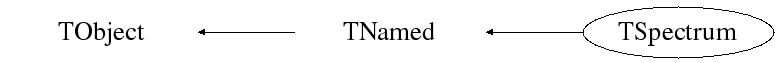## Function documentation

TSpectrum(const TSpectrum& )
``` Constructor.
```
TSpectrum(Int_t maxpositions, Float_t resolution = 1)
```  maxpositions:  maximum number of peaks
resolution:    determines resolution of the neighboring peaks
default value is 1 correspond to 3 sigma distance
between peaks. Higher values allow higher resolution
(smaller distance between peaks.
May be set later through SetResolution.
```

``` Destructor.
```
void SetAverageWindow(Int_t w = 3)
``` static function: Set average window of searched peaks
see TSpectrum::SearchHighRes
```
void SetDeconIterations(Int_t n = 3)
``` static function: Set max number of decon iterations in deconvolution operation
see TSpectrum::SearchHighRes
```
TH1 * Background(const TH1* hist, Int_t niter = 20, Option_t* option = "")
```   ONE-DIMENSIONAL BACKGROUND ESTIMATION FUNCTION
This function calculates the background spectrum in the input histogram h.
The background is returned as a histogram.

Function parameters:
-h: input 1-d histogram
-numberIterations, (default value = 20)
Increasing numberIterations make the result smoother and lower.
-option: may contain one of the following options
- to set the direction parameter
"BackIncreasingWindow". By default the direction is BackDecreasingWindow
- filterOrder-order of clipping filter,  (default "BackOrder2"
-possible values= "BackOrder4"
"BackOrder6"
"BackOrder8"
- "nosmoothing"- if selected, the background is not smoothed
By default the background is smoothed.
- smoothWindow-width of smoothing window, (default is "BackSmoothing3")
-possible values= "BackSmoothing5"
"BackSmoothing7"
"BackSmoothing9"
"BackSmoothing11"
"BackSmoothing13"
"BackSmoothing15"
- "Compton" if selected the estimation of Compton edge
will be included.
- "same" : if this option is specified, the resulting background
histogram is superimposed on the picture in the current pad.

NOTE that the background is only evaluated in the current range of h.
ie, if h has a bin range (set via h->GetXaxis()->SetRange(binmin,binmax),
the returned histogram will be created with the same number of bins
as the input histogram h, but only bins from binmin to binmax will be filled
with the estimated background.
```
void Print(Option_t* option = "") const
``` Print the array of positions
```
Int_t Search(const TH1* hist, Double_t sigma = 2, Option_t* option = "", Double_t threshold = 0.05)
```ONE-DIMENSIONAL PEAK SEARCH FUNCTION
This function searches for peaks in source spectrum in hin
The number of found peaks and their positions are written into
the members fNpeaks and fPositionX.
The search is performed in the current histogram range.

Function parameters:
hin:       pointer to the histogram of source spectrum
sigma:   sigma of searched peaks, for details we refer to manual
threshold: (default=0.05)  peaks with amplitude less than

By default, the background is removed before deconvolution.
Specify the option "nobackground" to not remove the background.       //

By default the "Markov" chain algorithm is used.
Specify the option "noMarkov" to disable this algorithm
Note that by default the source spectrum is replaced by a new spectrum//

By default a polymarker object is created and added to the list of
functions of the histogram. The histogram is drawn with the specified
option and the polymarker object drawn on top of the histogram.
The polymarker coordinates correspond to the npeaks peaks found in
the histogram.
A pointer to the polymarker object can be retrieved later via:
TList *functions = hin->GetListOfFunctions();
TPolyMarker *pm = (TPolyMarker*)functions->FindObject("TPolyMarker")
Specify the option "goff" to disable the storage and drawing of the
polymarker.

```
void SetResolution(Float_t resolution = 1)
```  resolution: determines resolution of the neighboring peaks
default value is 1 correspond to 3 sigma distance
between peaks. Higher values allow higher resolution
(smaller distance between peaks.
May be set later through SetResolution.
```
const char * Background(float* spectrum, Int_t ssize, Int_t numberIterations, Int_t direction, Int_t filterOrder, bool smoothing, Int_t smoothWindow, bool compton)
```        ONE-DIMENSIONAL BACKGROUND ESTIMATION FUNCTION - GENERAL FUNCTION

This function calculates background spectrum from source spectrum.
The result is placed in the vector pointed by spe1945ctrum pointer.

Function parameters:
spectrum-pointer to the vector of source spectrum
ssize-length of the spectrum vector
numberIterations-maximal width of clipping window,
direction- direction of change of clipping window
- possible values=kBackIncreasingWindow
kBackDecreasingWindow
filterOrder-order of clipping filter,
-possible values=kBackOrder2
kBackOrder4
kBackOrder6
kBackOrder8
smoothing- logical variable whether the smoothing operation
in the estimation of background will be included
- possible values=kFALSE
kTRUE
smoothWindow-width of smoothing window,
-possible values=kBackSmoothing3
kBackSmoothing5
kBackSmoothing7
kBackSmoothing9
kBackSmoothing11
kBackSmoothing13
kBackSmoothing15
compton- logical variable whether the estimation of Compton edge
will be included
- possible values=kFALSE
kTRUE

```

Background estimation

Goal: Separation of useful information (peaks) from useless information (background)

         method is based on Sensitive Nonlinear Iterative Peak (SNIP) clipping algorithm

          new value in the channel i is calculatedwhere p = 1, 2, , numberIterations. In fact it represents second order difference filter (-1,2,-1).

Function:

const char* Background(float *spectrum, int ssize, int numberIterations, int direction, int filterOrder,  bool smoothing,  int smoothingWindow, bool compton)

This function calculates background spectrum from the source spectrum.  The result is placed in the vector pointed by spectrum pointer.  One can also change the direction of the change of the clipping window, the order of the clipping filter, to include smoothing, to set width of smoothing window and to include the estimation of Compton edges. On successful completion it returns 0. On error it returns pointer to the string describing error.

Parameters:

spectrum-pointer to the vector of source spectrum

ssize-length of the spectrum vector

numberIterations-maximal width of clipping window,

direction- direction of change of clipping window

- possible values=kBackIncreasingWindow

kBackDecreasingWindow

filterOrder-order of clipping filter,

-possible values=kBackOrder2

kBackOrder4

kBackOrder6

kBackOrder8

smoothing- logical variable whether the smoothing operation in the estimation of

background will be included

- possible values=kFALSE

kTRUE

smoothWindow-width of smoothing window,

-possible values=kBackSmoothing3

kBackSmoothing5

kBackSmoothing7

kBackSmoothing9

kBackSmoothing11

kBackSmoothing13

kBackSmoothing15

compton- logical variable whether the estimation of Compton edge   will be included

- possible values=kFALSE

kTRUE

References:

  C. G Ryan et al.: SNIP, a statistics-sensitive background treatment for the quantitative analysis of PIXE spectra in geoscience applications. NIM, B34 (1988), 396-402.

  M. Morháč, J. Kliman, V. Matouek, M. Veselský, I. Turzo.: Background elimination methods for multidimensional gamma-ray spectra. NIM, A401 (1997) 113-132.

 D. D. Burgess, R. J. Tervo: Background estimation for gamma-ray spectroscopy. NIM 214 (1983), 431-434.

```
```

Example 1 script Background_incr.c :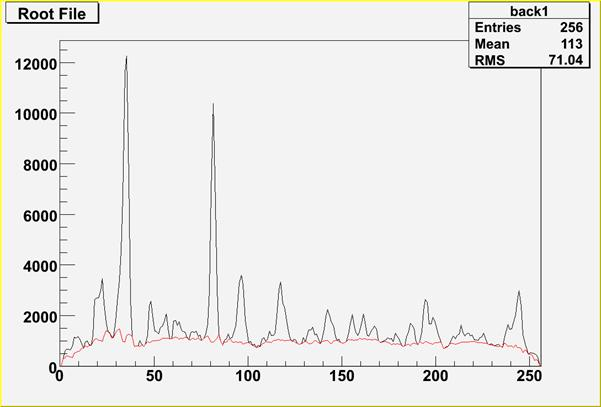Figure 1 Example of the estimation of background for number of iterations=6. Original spectrum is shown in black color, estimated background in red color.

Script:

// Example to illustrate the background estimator (class TSpectrum).

// To execute this example, do

// root > .x Background_incr.C

#include <TSpectrum>

void Background_incr() {

Int_t i;

Double_t nbins = 256;

Double_t xmin  = 0;

Double_t xmax  = (Double_t)nbins;

Float_t * source = new float[nbins];

TH1F *back = new TH1F("back","",nbins,xmin,xmax);

TH1F *d = new TH1F("d","",nbins,xmin,xmax);

TFile *f = new TFile("spectra\\TSpectrum.root");

back=(TH1F*) f->Get("back1;1");

TCanvas *Background = gROOT->GetListOfCanvases()->FindObject("Background");

if (!Background) Background = new TCanvas("Background","Estimation of background with increasing window",10,10,1000,700);

back->Draw("L");

TSpectrum *s = new TSpectrum();

for (i = 0; i < nbins; i++) source[i]=back->GetBinContent(i + 1);

s->Background(source,nbins,6,kBackIncreasingWindow,kBackOrder2,kFALSE,kBackSmoothing3,kFALSE);

for (i = 0; i < nbins; i++) d->SetBinContent(i + 1,source[i]);

d->SetLineColor(kRed);

d->Draw("SAME L");

}

```
```

Example 2  script Background_decr.c :

In Figure 1. one can notice that at the edges of the peaks the estimated background goes under the peaks. An alternative approach is to decrease the clipping window from a given value numberIterations to the value of one, which is presented in this example.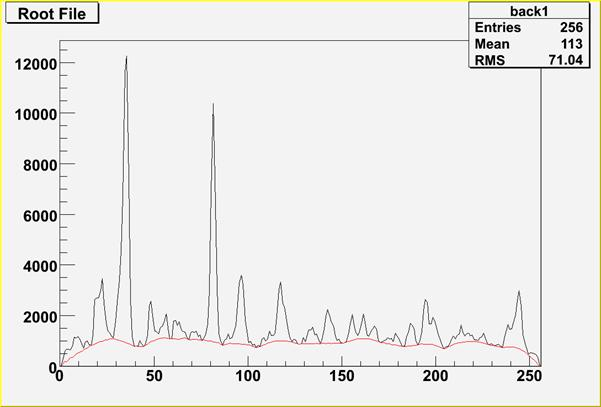Figure 2 Example of the estimation of background for numberIterations=6 using decreasing clipping window algorithm. Original spectrum is shown in black color, estimated background in red color.

Script:

// Example to illustrate the background estimator (class TSpectrum).

// To execute this example, do

// root > .x Background_decr.C

#include <TSpectrum>

void Background_decr() {

Int_t i;

Double_t nbins = 256;

Double_t xmin  = 0;

Double_t xmax  = (Double_t)nbins;

Float_t * source = new float[nbins];

TH1F *back = new TH1F("back","",nbins,xmin,xmax);

TH1F *d = new TH1F("d","",nbins,xmin,xmax);

TFile *f = new TFile("spectra\\TSpectrum.root");

back=(TH1F*) f->Get("back1;1");

TCanvas *Background = gROOT->GetListOfCanvases()->FindObject("Background");

if (!Background) Background = new TCanvas("Background","Estimation of background with decreasing window",10,10,1000,700);

back->Draw("L");

TSpectrum *s = new TSpectrum();

for (i = 0; i < nbins; i++) source[i]=back->GetBinContent(i + 1);

s->Background(source,nbins,6,kBackDecreasingWindow,kBackOrder2,kFALSE,kBackSmoothing3,kFALSE);

for (i = 0; i < nbins; i++) d->SetBinContent(i + 1,source[i]);

d->SetLineColor(kRed);

d->Draw("SAME L");

}

```
```

Example 3  script Background_width.c :

         the question is how to choose the width of the clipping window, i.e.,  numberIterations   parameter. The influence of this parameter on the estimated background is illustrated in Figure 3.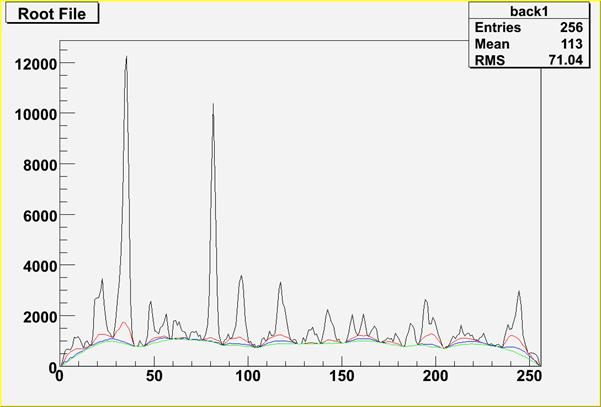Figure 3 Example of the influence of clipping window width on the estimated background for numberIterations=4 (red line), 6 (blue line) 8 (green line) using decreasing clipping window algorithm.

        in general one should set this parameter so that the value 2*numberIterations+1 was greater than the widths of preserved objects (peaks).

Script:

// Example to illustrate the influence of the clipping window width on the estimated background

// To execute this example, do

// root > .x Background_width.C

#include <TSpectrum>

void Background_width() {

Int_t i;

Double_t nbins = 256;

Double_t xmin  = 0;

Double_t xmax  = (Double_t)nbins;

Float_t * source = new float[nbins];

TH1F *h = new TH1F("h","",nbins,xmin,xmax);

TH1F *d1 = new TH1F("d1","",nbins,xmin,xmax);

TH1F *d2 = new TH1F("d2","",nbins,xmin,xmax);

TH1F *d3 = new TH1F("d3","",nbins,xmin,xmax);

TFile *f = new TFile("spectra\\TSpectrum.root");

h=(TH1F*) f->Get("back1;1");

TCanvas *background = gROOT->GetListOfCanvases()->FindObject("background");

if (!background) background = new TCanvas("background","Influence of clipping window width on the estimated background",10,10,1000,700);

h->Draw("L");

TSpectrum *s = new TSpectrum();

for (i = 0; i < nbins; i++) source[i]=h->GetBinContent(i + 1);

s->Background(source,nbins,4,kBackDecreasingWindow,kBackOrder2,kFALSE,kBackSmoothing3,kFALSE);

for (i = 0; i < nbins; i++) d1->SetBinContent(i + 1,source[i]);

d1->SetLineColor(kRed);

d1->Draw("SAME L");

for (i = 0; i < nbins; i++) source[i]=h->GetBinContent(i + 1);

s->Background(source,nbins,6,kBackDecreasingWindow,kBackOrder2,kFALSE,kBackSmoothing3,kFALSE);

for (i = 0; i < nbins; i++) d2->SetBinContent(i + 1,source[i]);

d2->SetLineColor(kBlue);

d2->Draw("SAME L");

for (i = 0; i < nbins; i++) source[i]=h->GetBinContent(i + 1);

s->Background(source,nbins,8,kBackDecreasingWindow,kBackOrder2,kFALSE,kBackSmoothing3,kFALSE);

for (i = 0; i < nbins; i++) d3->SetBinContent(i + 1,source[i]);

d3->SetLineColor(kGreen);

d3->Draw("SAME L");

}

```
```

Example 4  script Background_width2.c :

         another example for very complex spectrum is given in Figure 4.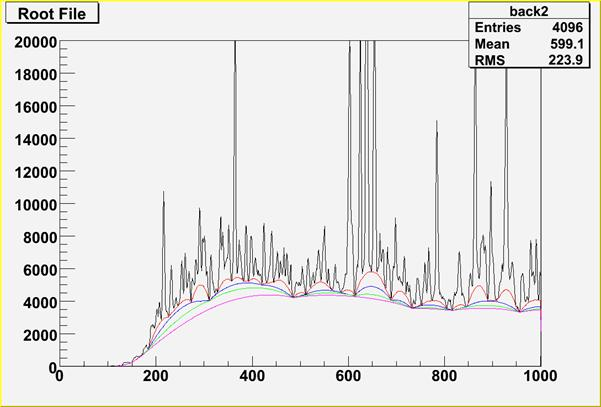Figure 4 Example of the influence of clipping window width on the estimated background for numberIterations=10 (red line), 20 (blue line), 30 (green line) and 40 (magenta line) using decreasing clipping window algorithm.

Script:

// Example to illustrate the influence of the clipping window width on the estimated background

// To execute this example, do

// root > .x Background_width2.C

#include <TSpectrum>

void Background_width2() {

Int_t i;

Double_t nbins = 4096;

Double_t xmin  = 0;

Double_t xmax  = (Double_t)4096;

Float_t * source = new float[nbins];

TH1F *h = new TH1F("h","",nbins,xmin,xmax);

TH1F *d1 = new TH1F("d1","",nbins,xmin,xmax);

TH1F *d2 = new TH1F("d2","",nbins,xmin,xmax);

TH1F *d3 = new TH1F("d3","",nbins,xmin,xmax);

TH1F *d4 = new TH1F("d4","",nbins,xmin,xmax);

TFile *f = new TFile("spectra\\TSpectrum.root");

h=(TH1F*) f->Get("back2;1");

TCanvas *background = gROOT->GetListOfCanvases()->FindObject("background");

if (!background) background = new TCanvas("background","Influence of clipping window width on the estimated background",10,10,1000,700);

h->SetAxisRange(0,1000);

h->SetMaximum(20000);

h->Draw("L");

TSpectrum *s = new TSpectrum();

for (i = 0; i < nbins; i++) source[i]=h->GetBinContent(i + 1);

s->Background(source,nbins,10,kBackDecreasingWindow,kBackOrder2,kFALSE,kBackSmoothing3,kFALSE);

for (i = 0; i < nbins; i++) d1->SetBinContent(i + 1,source[i]);

d1->SetLineColor(kRed);

d1->Draw("SAME L");

for (i = 0; i < nbins; i++) source[i]=h->GetBinContent(i + 1);

s->Background(source,nbins,20,kBackDecreasingWindow,kBackOrder2,kFALSE,kBackSmoothing3,kFALSE);

for (i = 0; i < nbins; i++) d2->SetBinContent(i + 1,source[i]);

d2->SetLineColor(kBlue);

d2->Draw("SAME L");

for (i = 0; i < nbins; i++) source[i]=h->GetBinContent(i + 1);

s->Background(source,nbins,30,kBackDecreasingWindow,kBackOrder2,kFALSE,kBackSmoothing3,kFALSE);

for (i = 0; i < nbins; i++) d3->SetBinContent(i + 1,source[i]);

d3->SetLineColor(kGreen);

d3->Draw("SAME L");

for (i = 0; i < nbins; i++) source[i]=h->GetBinContent(i + 1);

s->Background(source,nbins,10,kBackDecreasingWindow,kBackOrder2,kFALSE,kBackSmoothing3,kFALSE);

for (i = 0; i < nbins; i++) d4->SetBinContent(i + 1,source[i]);

d4->SetLineColor(kMagenta);

d4->Draw("SAME L");

}

```
```

Example 5  script Background_order.c :

         second order difference filter removes linear (quasi-linear) background and preserves symmetrical peaks.

         however if the shape of the background is more complex one can employ higher-order clipping filters (see example in Figure 5)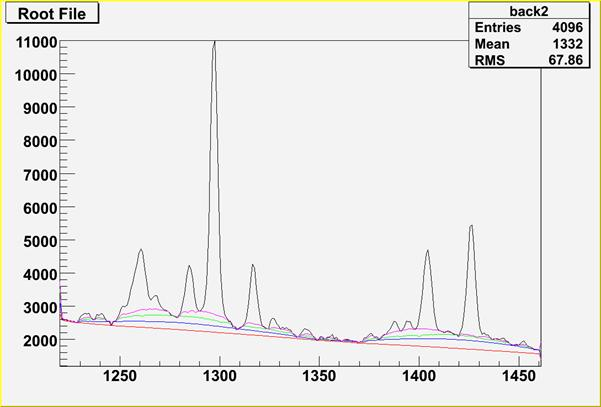Figure 5 Example of the influence of clipping filter difference order on the estimated background for fNnumberIterations=40, 2-nd order red line, 4-th order blue line, 6-th order green line and 8-th order magenta line, and using decreasing clipping window algorithm.

Script:

// Example to illustrate the influence of the clipping filter difference order on the estimated background

// To execute this example, do

// root > .x Background_order.C

#include <TSpectrum>

void Background_order() {

Int_t i;

Double_t nbins = 4096;

Double_t xmin  = 0;

Double_t xmax  = (Double_t)4096;

Float_t * source = new float[nbins];

TH1F *h = new TH1F("h","",nbins,xmin,xmax);

TH1F *d1 = new TH1F("d1","",nbins,xmin,xmax);

TH1F *d2 = new TH1F("d2","",nbins,xmin,xmax);

TH1F *d3 = new TH1F("d3","",nbins,xmin,xmax);

TH1F *d4 = new TH1F("d4","",nbins,xmin,xmax);

TFile *f = new TFile("spectra\\TSpectrum.root");

h=(TH1F*) f->Get("back2;1");

TCanvas *background = gROOT->GetListOfCanvases()->FindObject("background");

if (!background) background = new TCanvas("background","Influence of clipping filter difference order on the estimated background",10,10,1000,700);

h->SetAxisRange(1220,1460);

h->SetMaximum(11000);

h->Draw("L");

TSpectrum *s = new TSpectrum();

for (i = 0; i < nbins; i++) source[i]=h->GetBinContent(i + 1);

s->Background(source,nbins,40,kBackDecreasingWindow,kBackOrder2,kFALSE,kBackSmoothing3,kFALSE);

for (i = 0; i < nbins; i++) d1->SetBinContent(i + 1,source[i]);

d1->SetLineColor(kRed);

d1->Draw("SAME L");

for (i = 0; i < nbins; i++) source[i]=h->GetBinContent(i + 1);

s->Background(source,nbins,40,kBackDecreasingWindow,kBackOrder4,kFALSE,kBackSmoothing3,kFALSE);

for (i = 0; i < nbins; i++) d2->SetBinContent(i + 1,source[i]);

d2->SetLineColor(kBlue);

d2->Draw("SAME L");

for (i = 0; i < nbins; i++) source[i]=h->GetBinContent(i + 1);

s->Background(source,nbins,40,kBackDecreasingWindow,kBackOrder6,kFALSE,kBackSmoothing3,kFALSE);

for (i = 0; i < nbins; i++) d3->SetBinContent(i + 1,source[i]);

d3->SetLineColor(kGreen);

d3->Draw("SAME L");

for (i = 0; i < nbins; i++) source[i]=h->GetBinContent(i + 1);

s->Background(source,nbins,40,kBackDecreasingWindow,kBackOrder8,kFALSE,kBackSmoothing3,kFALSE);

for (i = 0; i < nbins; i++) d4->SetBinContent(i + 1,source[i]);

d4->SetLineColor(kMagenta);

d4->Draw("SAME L");

}

```
```

Example 6  script Background_smooth.c :

         the estimate of the background can be influenced by noise present in the spectrum. We proposed  the algorithm of the background estimate with simultaneous smoothing

         in the original algorithm without smoothing, the estimated background snatches the lower spikes in the noise. Consequently, the areas of peaks are biased by this error.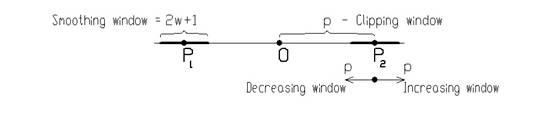Figure 7 Principle of background estimation algorithm with  simultaneous smoothing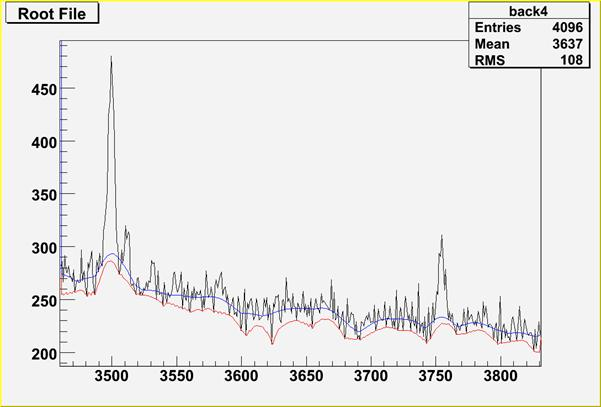Figure 8 Illustration of non-smoothing (red line) and smoothing algorithm of background estimation (blue line).

Script:

// Example to illustrate the background estimator (class TSpectrum) including Compton edges.

// To execute this example, do

// root > .x Background_smooth.C

#include <TSpectrum>

void Background_smooth() {

Int_t i;

Double_t nbins = 4096;

Double_t xmin  = 0;

Double_t xmax  = (Double_t)nbins;

Float_t * source = new float[nbins];

TH1F *h = new TH1F("h","",nbins,xmin,xmax);

TH1F *d1 = new TH1F("d1","",nbins,xmin,xmax);

TH1F *d2 = new TH1F("d2","",nbins,xmin,xmax);

TFile *f = new TFile("spectra\\TSpectrum.root");

h=(TH1F*) f->Get("back4;1");

TCanvas *background = gROOT->GetListOfCanvases()->FindObject("background");

if (!background) background = new TCanvas("background","Estimation of background with noise",10,10,1000,700);

h->SetAxisRange(3460,3830);

h->Draw("L");

TSpectrum *s = new TSpectrum();

for (i = 0; i < nbins; i++) source[i]=h->GetBinContent(i + 1);

s->Background(source,nbins,6,kBackDecreasingWindow,kBackOrder2,kFALSE,kBackSmoothing3,kFALSE);

for (i = 0; i < nbins; i++) d1->SetBinContent(i + 1,source[i]);

d1->SetLineColor(kRed);

d1->Draw("SAME L");

for (i = 0; i < nbins; i++) source[i]=h->GetBinContent(i + 1);

s->Background(source,nbins,6,kBackDecreasingWindow,kBackOrder2,kTRUE,kBackSmoothing3,kFALSE);

for (i = 0; i < nbins; i++) d2->SetBinContent(i + 1,source[i]);

d2->SetLineColor(kBlue);

d2->Draw("SAME L");

}

```
```

Example 8  script Background_compton.c :

         sometimes it is necessary to include also the Compton edges into the estimate of the background. In Figure 8 we present the example of the synthetic spectrum with Compton edges.

         the background was estimated using the 8-th order filter with the estimation of the Compton edges using decreasing clipping window algorithm (numberIterations=10) with smoothing ( smoothingWindow=5).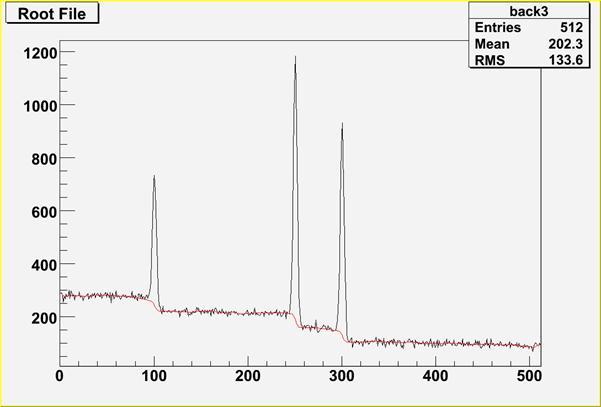Figure 8 Example of the estimate of the background with Compton edges (red line) for numberIterations=10, 8-th order difference filter, using decreasing clipping window algorithm and smoothing (smoothingWindow=5).

Script:

// Example to illustrate the background estimator (class TSpectrum) including Compton edges.

// To execute this example, do

// root > .x Background_compton.C

#include <TSpectrum>

void Background_compton() {

Int_t i;

Double_t nbins = 512;

Double_t xmin  = 0;

Double_t xmax  = (Double_t)nbins;

Float_t * source = new float[nbins];

TH1F *h = new TH1F("h","",nbins,xmin,xmax);

TH1F *d1 = new TH1F("d1","",nbins,xmin,xmax);

TFile *f = new TFile("spectra\\TSpectrum.root");

h=(TH1F*) f->Get("back3;1");

TCanvas *background = gROOT->GetListOfCanvases()->FindObject("background");

if (!background) background = new TCanvas("background","Estimation of background with Compton edges under peaks",10,10,1000,700);

h->Draw("L");

TSpectrum *s = new TSpectrum();

for (i = 0; i < nbins; i++) source[i]=h->GetBinContent(i + 1);

s->Background(source,nbins,10,kBackDecreasingWindow,kBackOrder8,kTRUE,kBackSmoothing5,,kTRUE);

for (i = 0; i < nbins; i++) d1->SetBinContent(i + 1,source[i]);

d1->SetLineColor(kRed);

d1->Draw("SAME L");

}

const char* SmoothMarkov(float* source, Int_t ssize, Int_t averWindow)
```        ONE-DIMENSIONAL MARKOV SPECTRUM SMOOTHING FUNCTION

This function calculates smoothed spectrum from source spectrum
based on Markov chain method.
The result is placed in the array pointed by source pointer.

Function parameters:
source-pointer to the array of source spectrum
ssize-length of source array
averWindow-width of averaging smoothing window

```

Smoothing

Goal: Suppression of statistical fluctuations

         the algorithm is based on discrete Markov chain, which has very simple invariant distribution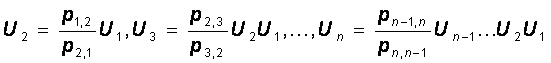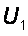being defined from the normalization condition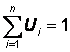n is the length of the smoothed spectrum and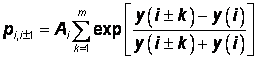is the probability of the change of the peak position from channel i to the channel i+1.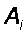is the normalization constant so that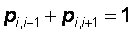and m is a width of smoothing window.

Function:

const char* SmoothMarkov(float *spectrum, int ssize,  int averWindow)

This function calculates smoothed spectrum from the source spectrum based on Markov chain method. The result is placed in the vector pointed by source pointer. On successful completion it returns 0. On error it returns pointer to the string describing error.

Parameters:

spectrum-pointer to the vector of source spectrum

ssize-length of the spectrum vector

averWindow-width of averaging smoothing window

Reference:

 Z.K. Silagadze, A new algorithm for automatic photopeak searches. NIM A 376 (1996), 451.

```
```

Example 14  script Smoothing.c :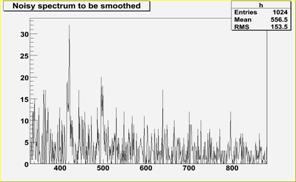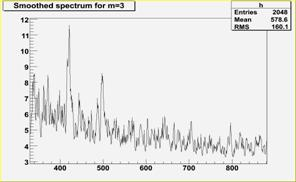Fig. 23 Original noisy spectrum    Fig. 24 Smoothed spectrum m=3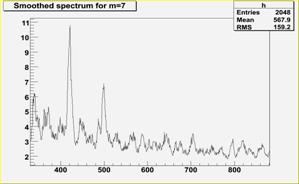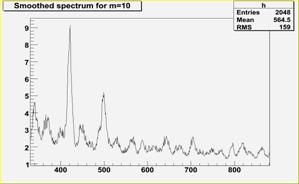Fig. 25 Smoothed spectrum m=7 Fig.26 Smoothed spectrum m=10

Script:

// Example to illustrate smoothing using Markov algorithm (class TSpectrum).

// To execute this example, do

// root > .x Smoothing.C

//#include <TSpectrum>

void Smoothing() {

Int_t i;

Double_t nbins = 1024;

Double_t xmin  = 0;

Double_t xmax  = (Double_t)nbins;

Float_t * source = new float[nbins];

TH1F *h = new TH1F("h","Smoothed spectrum for m=3",nbins,xmin,xmax);

TFile *f = new TFile("spectra\\TSpectrum.root");

h=(TH1F*) f->Get("smooth1;1");

for (i = 0; i < nbins; i++) source[i]=h->GetBinContent(i + 1);

TCanvas *Smooth1 = gROOT->GetListOfCanvases()->FindObject("Smooth1");

if (!Smooth1) Smooth1 = new TCanvas("Smooth1","Smooth1",10,10,1000,700);

TSpectrum *s = new TSpectrum();

s->SmoothMarkov(source,1024,3);  //3, 7, 10

for (i = 0; i < nbins; i++) h->SetBinContent(i + 1,source[i]);

h->SetAxisRange(330,880);

h->Draw("L");

}

const char * Deconvolution(float* source, const float* response, Int_t ssize, Int_t numberIterations, Int_t numberRepetitions, Double_t boost)
```ONE-DIMENSIONAL DECONVOLUTION FUNCTION
This function calculates deconvolution from source spectrum
according to response spectrum using Gold algorithm
The result is placed in the vector pointed by source pointer.

Function parameters:
source:  pointer to the vector of source spectrum
response:     pointer to the vector of response spectrum
ssize:    length of source and response spectra
numberIterations, for details we refer to the reference given below
umberRepetitions, for repeated boosted deconvolutio
boost, boosting coefficient

M. Morhac, J. Kliman, V. Matousek, M. Veselský, I. Turzo.:
Efficient one- and two-dimensional Gold deconvolution and its
application to gamma-ray spectra decomposition.
NIM, A401 (1997) 385-408.

```

Deconvolution

Goal: Improvement of the resolution in spectra, decomposition of multiplets

Mathematical formulation of the convolution system iswhere h(i) is the impulse response function, x, y are input and output vectors, respectively, N is the length of x and h vectors. In matrix form we have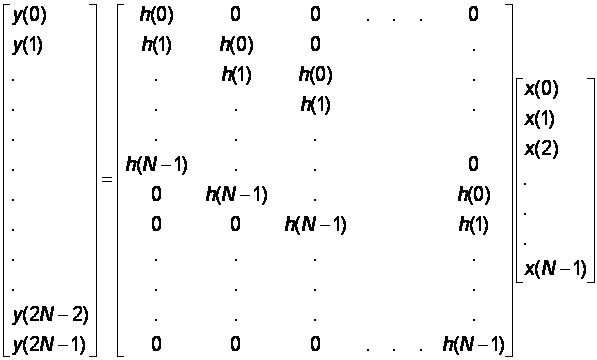         let us assume that we know the response and the output vector (spectrum) of the above given system.

         the deconvolution represents solution of the overdetermined system of linear equations, i.e.,  the calculation of the vector x.

         from numerical stability point of view the operation of deconvolution is extremely critical (ill-posed  problem) as well as time consuming operation.

         the Gold deconvolution algorithm proves to work very well, other methods (Fourier, VanCittert etc) oscillate.

         it is suitable to process positive definite data (e.g. histograms).

Gold deconvolution algorithmwhere L is given number of iterations (numberIterations parameter).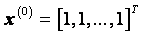Boosted deconvolution

1.    Set the initial solution

2.    Set required number of repetitions R and iterations L

3.    Set r = 1.

4.    Using Gold deconvolution algorithm for k=1,2,...,L  find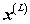5.    If  r = R stop calculation, else

a. apply boosting operation, i.e., seti=0,1,...N-1 and p is boosting coefficient >0.

b. r = r + 1

c. continue in 4.

Function:

const char* Deconvolution(float *source, const float *respMatrix, int ssize, int numberIterations, int numberRepetitions, double boost)

This function calculates deconvolution from source spectrum according to response spectrum using Gold deconvolution algorithm. The result is placed in the vector pointed by source pointer. On successful completion it returns 0. On error it returns pointer to the string describing error. If desired after every numberIterations one can apply boosting operation (exponential function with exponent given by boost coefficient) and repeat it numberRepetitions times.

Parameters:

source-pointer to the vector of source spectrum

respMatrix-pointer to the vector of response spectrum

ssize-length of the spectrum vector

numberIterations-number of iterations (parameter l in the Gold deconvolution

algorithm)

numberRepetitions-number of repetitions for boosted deconvolution. It must be

greater or equal to one.

boost-boosting coefficient, applies only if numberRepetitions is greater than one.

Recommended range <1,2>.

References:

 Gold R., ANL-6984, Argonne National Laboratories, Argonne Ill, 1964.

 Coote G.E., Iterative smoothing and deconvolution of one- and two-dimensional elemental distribution data, NIM B 130 (1997) 118.

 M. Morháč, J. Kliman, V. Matouek, M. Veselský, I. Turzo.: Efficient one- and two-dimensional Gold deconvolution and its application to gamma-ray spectra decomposition. NIM, A401 (1997) 385-408.

 Morháč M., Matouek V., Kliman J., Efficient algorithm of multidimensional deconvolution and its application to nuclear data processing, Digital Signal Processing 13 (2003) 144.

```
```

Example 8  script Deconvolution.c :

         response function (usually peak) should be shifted left to the first non-zero channel (bin) (see Figure 9)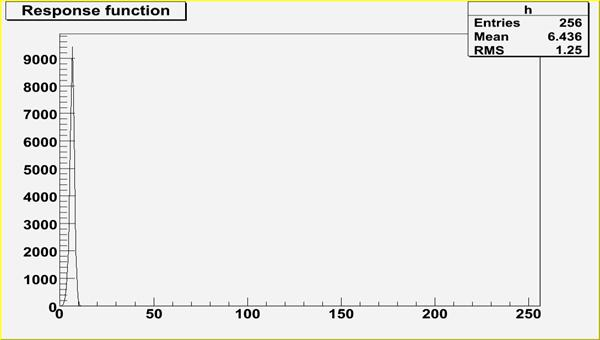Figure 9 Response spectrum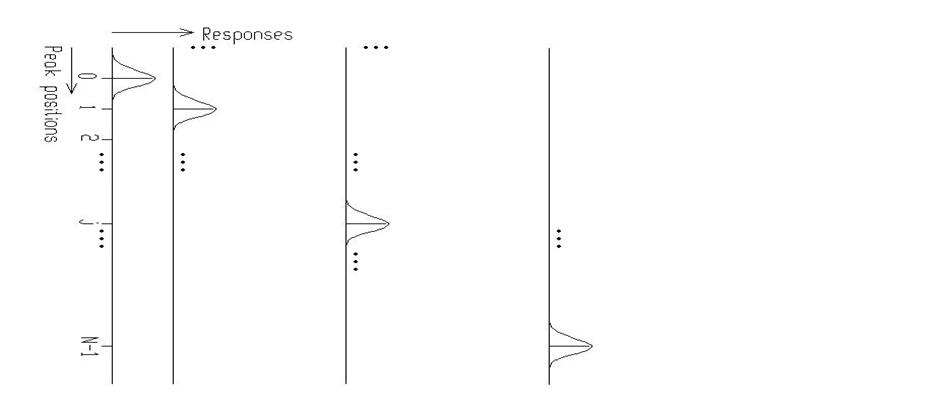Figure 10 Principle how the response matrix is composed inside of the Deconvolution function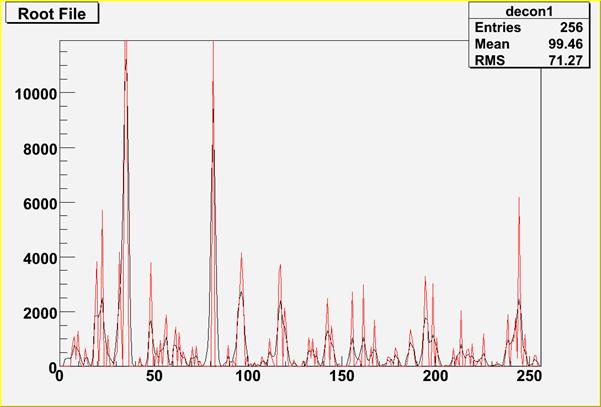Figure 11 Example of Gold deconvolution. The original source spectrum is drawn with black color, the spectrum after the deconvolution (10000 iterations) with red color

Script:

// Example to illustrate deconvolution function (class TSpectrum).

// To execute this example, do

// root > .x Deconvolution.C

#include <TSpectrum>

void Deconvolution() {

Int_t i;

Double_t nbins = 256;

Double_t xmin  = 0;

Double_t xmax  = (Double_t)nbins;

Float_t * source = new float[nbins];

Float_t * response = new float[nbins];

TH1F *h = new TH1F("h","Deconvolution",nbins,xmin,xmax);

TH1F *d = new TH1F("d","",nbins,xmin,xmax);

TFile *f = new TFile("spectra\\TSpectrum.root");

h=(TH1F*) f->Get("decon1;1");

TFile *fr = new TFile("spectra\\TSpectrum.root");

d=(TH1F*) fr->Get("decon_response;1");

for (i = 0; i < nbins; i++) source[i]=h->GetBinContent(i + 1);

for (i = 0; i < nbins; i++) response[i]=d->GetBinContent(i + 1);

TCanvas *Decon1 = gROOT->GetListOfCanvases()->FindObject("Decon1");

if (!Decon1) Decon1 = new TCanvas("Decon1","Decon1",10,10,1000,700);

h->Draw("L");

TSpectrum *s = new TSpectrum();

s->Deconvolution(source,response,256,1000,1,1);

for (i = 0; i < nbins; i++) d->SetBinContent(i + 1,source[i]);

d->SetLineColor(kRed);

d->Draw("SAME L");

}

```
```

Examples of Gold deconvolution method

         first let us study the influence of the number of iterations on the deconvolved spectrum  (Figure 12)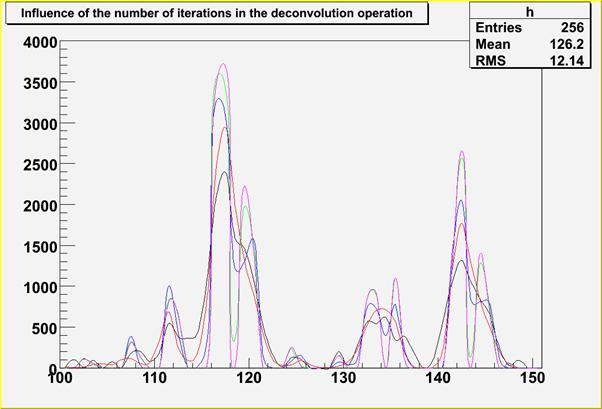Figure 12 Study of Gold deconvolution algorithm. The original source spectrum is drawn with black color, spectrum after 100 iterations with red color, spectrum after 1000 iterations with blue color, spectrum after 10000 iterations with green color and  spectrum after 100000 iterations with magenta color.

      for relatively narrow peaks in the above given example the Gold deconvolution method is able to decompose  overlapping peaks practically to delta - functions.

         in the next example we have chosen a synthetic data (spectrum, 256 channels) consisting of 5 very closely positioned, relatively wide peaks (sigma =5), with added noise (Figure 13).

         thin lines represent pure Gaussians (see Table 1); thick line is a resulting spectrum with additive noise (10% of the amplitude of small peaks).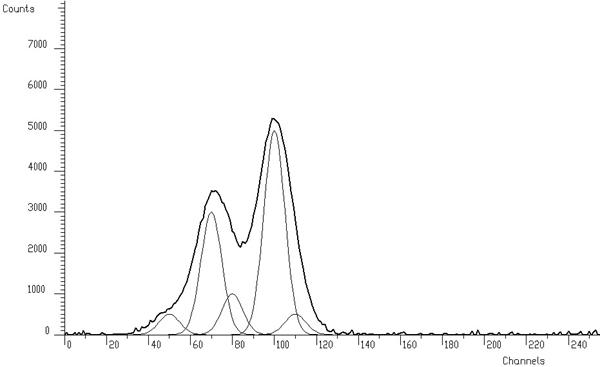Figure 13 Testing example of synthetic spectrum composed of 5 Gaussians with added noise

 Peak # Position Height Area 1 50 500 10159 2 70 3000 60957 3 80 1000 20319 4 100 5000 101596 5 110 500 10159

Table 1 Positions, heights and areas of peaks in the spectrum shown in Figure 13

         in ideal case, we should obtain the result given in Figure 14. The areas of the Gaussian components of the spectrum are concentrated completely to delta functions

         when solving the overdetermined system of linear equations with data from Figure 13 in the sense of minimum least squares criterion without any regularization we obtain the result with large oscillations  (Figure 15).

         from mathematical point of view, it is the optimal solution in the unconstrained space of independent variables. >From physical point of view we are interested only in a meaningful solution.

         therefore, we have to employ regularization techniques (e.g. Gold deconvolution) and/or to confine the space of allowed solutions to subspace of positive solutions.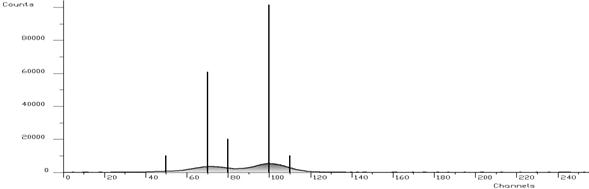Figure 14 The same spectrum like in Figure 13, outlined bars show the contents of present components (peaks)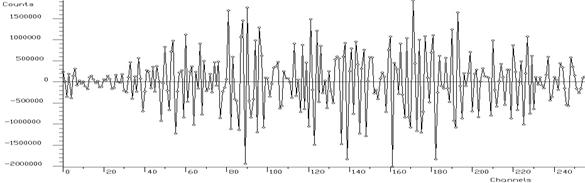Figure 15 Least squares solution of the system of linear equations without regularization

Example 9  script Deconvolution_wide.c :

         when we employ Gold deconvolution algorithm we obtain the result given in Fig. 16. One can observe that the resulting spectrum is smooth. On the other hand the method is not able to decompose completely the peaks in the spectrum.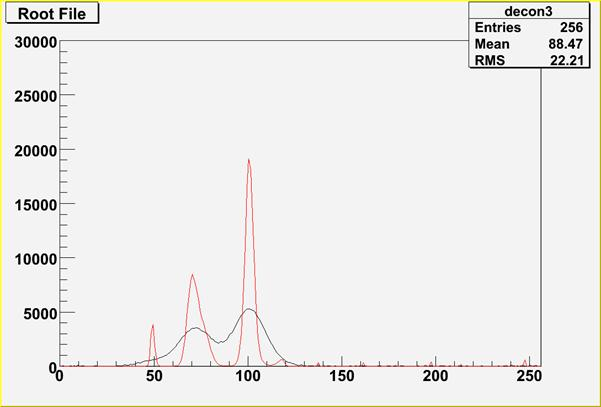Figure 16 Example of Gold deconvolution for closely positioned wide peaks. The original source spectrum is drawn with black color, the spectrum after the deconvolution (10000 iterations) with red color

Script:

// Example to illustrate deconvolution function (class TSpectrum).

// To execute this example, do

// root > .x Deconvolution_wide.C

#include <TSpectrum>

void Deconvolution_wide() {

Int_t i;

Double_t nbins = 256;

Double_t xmin  = 0;

Double_t xmax  = (Double_t)nbins;

Float_t * source = new float[nbins];

Float_t * response = new float[nbins];

TH1F *h = new TH1F("h","Deconvolution",nbins,xmin,xmax);

TH1F *d = new TH1F("d","",nbins,xmin,xmax);

TFile *f = new TFile("spectra\\TSpectrum.root");

h=(TH1F*) f->Get("decon3;1");

TFile *fr = new TFile("spectra\\TSpectrum.root");

d=(TH1F*) fr->Get("decon_response_wide;1");

for (i = 0; i < nbins; i++) source[i]=h->GetBinContent(i + 1);

for (i = 0; i < nbins; i++) response[i]=d->GetBinContent(i + 1);

TCanvas *Decon1 = gROOT->GetListOfCanvases()->FindObject("Decon1");

if (!Decon1) Decon1 = new TCanvas("Decon1","Deconvolution of closely positioned overlapping peaks using Gold deconvolution method",10,10,1000,700);

h->SetMaximum(30000);

h->Draw("L");

TSpectrum *s = new TSpectrum();

s->Deconvolution(source,response,256,10000,1,1);

for (i = 0; i < nbins; i++) d->SetBinContent(i + 1,source[i]);

d->SetLineColor(kRed);

d->Draw("SAME L");

}

Example 10  script Deconvolution_wide_boost.c :

         further let us employ boosting operation into deconvolution (Fig. 17)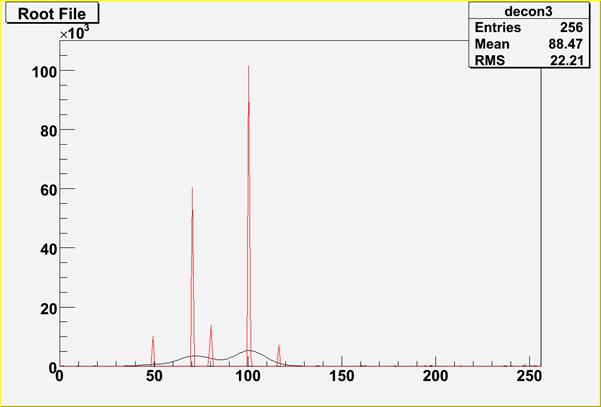Figure 17 The original source spectrum is drawn with black color, the spectrum after the deconvolution with red color. Number of iterations = 200, number of repetitions = 50 and boosting coefficient = 1.2.

 Peak # Original/Estimated (max) position Original/Estimated area 1 50/49 10159/10419 2 70/70 60957/58933 3 80/79 20319/19935 4 100/100 101596/105413 5 110/117 10159/6676

Table 2 Results of the estimation of peaks in spectrum shown in Figure 17

                     one can observe that peaks are decomposed practically to delta functions. Number of peaks is correct, positions of big peaks as well as their areas are relatively well estimated. However there is a considerable error in the estimation of the position of small right hand peak.

Script:

// Example to illustrate deconvolution function (class TSpectrum).

// To execute this example, do

// root > .x Deconvolution_wide_boost.C

#include <TSpectrum>

void Deconvolution_wide_boost() {

Int_t i;

Double_t nbins = 256;

Double_t xmin  = 0;

Double_t xmax  = (Double_t)nbins;

Float_t * source = new float[nbins];

Float_t * response = new float[nbins];

TH1F *h = new TH1F("h","Deconvolution",nbins,xmin,xmax);

TH1F *d = new TH1F("d","",nbins,xmin,xmax);

TFile *f = new TFile("spectra\\TSpectrum.root");

h=(TH1F*) f->Get("decon3;1");

TFile *fr = new TFile("spectra\\TSpectrum.root");

d=(TH1F*) fr->Get("decon_response_wide;1");

for (i = 0; i < nbins; i++) source[i]=h->GetBinContent(i + 1);

for (i = 0; i < nbins; i++) response[i]=d->GetBinContent(i + 1);

TCanvas *Decon1 = gROOT->GetListOfCanvases()->FindObject("Decon1");

if (!Decon1) Decon1 = new TCanvas("Decon1","Deconvolution of closely positioned overlapping peaks using boosted Gold deconvolution method",10,10,1000,700);

h->SetMaximum(110000);

h->Draw("L");

TSpectrum *s = new TSpectrum();

s->Deconvolution(source,response,256,200,50,1.2);

for (i = 0; i < nbins; i++) d->SetBinContent(i + 1,source[i]);

d->SetLineColor(kRed);

d->Draw("SAME L");

}

const char * DeconvolutionRL(float* source, const float* response, Int_t ssize, Int_t numberIterations, Int_t numberRepetitions, Double_t boost)
```ONE-DIMENSIONAL DECONVOLUTION FUNCTION
This function calculates deconvolution from source spectrum
according to response spectrum using Richardson-Lucy algorithm
The result is placed in the vector pointed by source pointer.

Function parameters:
source:  pointer to the vector of source spectrum
response:     pointer to the vector of response spectrum
ssize:    length of source and response spectra
numberIterations, for details we refer to the reference given above
umberRepetitions, for repeated boosted deconvolutio
boost, boosting coefficient

```

Richardson-Lucy deconvolution algorithm

·              for discrete systems it has the form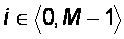·               for positive input data and response matrix this iterative method forces the deconvoluted spectra to be non-negative.

·              the Richardson-Lucy iteration converges to the maximum likelihood solution for Poisson statistics in the data.

Function:

const char* DeconvolutionRL(float *source, const float *respMatrix, int ssize, int numberIterations, int numberRepetitions, double boost)

This function calculates deconvolution from source spectrum according to response spectrum using Richardson-Lucy deconvolution algorithm. The result is placed in the vector pointed by source pointer. On successful completion it returns 0. On error it returns pointer to the string describing error. If desired after every numberIterations one can apply boosting operation (exponential function with exponent given by boost coefficient) and repeat it numberRepetitions times (see Gold deconvolution).

Parameters:

source-pointer to the vector of source spectrum

respMatrix-pointer to the vector of response spectrum

ssize-length of the spectrum vector

numberIterations-number of iterations (parameter l in the Gold deconvolution

algorithm)

numberRepetitions-number of repetitions for boosted deconvolution. It must be

greater or equal to one.

boost-boosting coefficient, applies only if numberRepetitions is greater than one.

Recommended range <1,2>.

References:

 Abreu M.C. et al., A four-dimensional deconvolution method to correct NA38 experimental data, NIM A 405 (1998) 139.

 Lucy L.B., A.J. 79 (1974) 745.

 Richardson W.H., J. Opt. Soc. Am. 62 (1972) 55.

```
```

Examples of Richardson-Lucy deconvolution method

Example 11  script DeconvolutionRL_wide.c :

         when we employ Richardson-Lucy deconvolution algorithm to our data from Fig. 13 we obtain the result given in Fig. 18. One can observe improvements as compared to the result achieved by Gold deconvolution.

         neverthless it is unable to decompose the multiplet.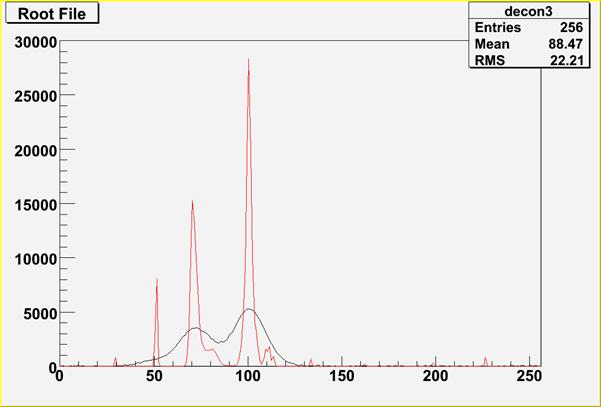Figure 18 Example of Richardson-Lucy deconvolution for closely positioned wide peaks. The original source spectrum is drawn with black color, the spectrum after the deconvolution (10000 iterations) with red color

Script:

// Example to illustrate deconvolution function (class TSpectrum).

// To execute this example, do

// root > .x DeconvolutionRL_wide.C

#include <TSpectrum>

void DeconvolutionRL_wide() {

Int_t i;

Double_t nbins = 256;

Double_t xmin  = 0;

Double_t xmax  = (Double_t)nbins;

Float_t * source = new float[nbins];

Float_t * response = new float[nbins];

TH1F *h = new TH1F("h","Deconvolution",nbins,xmin,xmax);

TH1F *d = new TH1F("d","",nbins,xmin,xmax);

TFile *f = new TFile("spectra\\TSpectrum.root");

h=(TH1F*) f->Get("decon3;1");

TFile *fr = new TFile("spectra\\TSpectrum.root");

d=(TH1F*) fr->Get("decon_response_wide;1");

for (i = 0; i < nbins; i++) source[i]=h->GetBinContent(i + 1);

for (i = 0; i < nbins; i++) response[i]=d->GetBinContent(i + 1);

TCanvas *Decon1 = gROOT->GetListOfCanvases()->FindObject("Decon1");

if (!Decon1) Decon1 = new TCanvas("Decon1","Deconvolution of closely positioned overlapping peaks using Richardson-Lucy deconvolution method",10,10,1000,700);

h->SetMaximum(30000);

h->Draw("L");

TSpectrum *s = new TSpectrum();

s->DeconvolutionRL(source,response,256,10000,1,1);

for (i = 0; i < nbins; i++) d->SetBinContent(i + 1,source[i]);

d->SetLineColor(kRed);

d->Draw("SAME L");

}

Example 12  script DeconvolutionRL_wide_boost.c :

         further let us employ boosting operation into deconvolution (Fig. 19)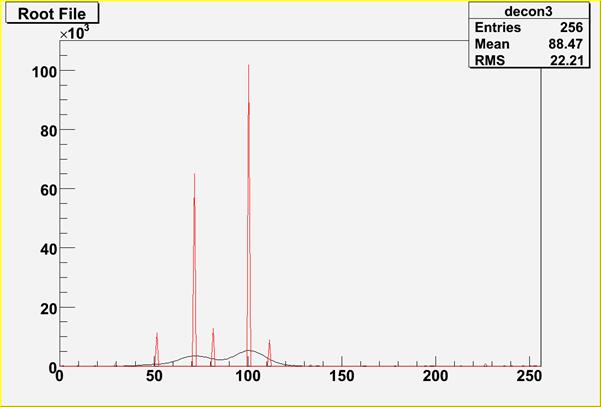Figure 19 The original source spectrum is drawn with black color, the spectrum after the deconvolution with red color. Number of iterations = 200, number of repetitions = 50 and boosting coefficient = 1.2.

 Peak # Original/Estimated (max) position Original/Estimated area 1 50/51 10159/11426 2 70/71 60957/65003 3 80/81 20319/12813 4 100/100 101596/101851 5 110/111 10159/8920

Table 3 Results of the estimation of peaks in spectrum shown in Figure 19

                     one can observe improvements in the estimation of peak positions as compared to the results achieved by Gold deconvolution

Script:

// Example to illustrate deconvolution function (class TSpectrum).

// To execute this example, do

// root > .x DeconvolutionRL_wide_boost.C

#include <TSpectrum>

void DeconvolutionRL_wide_boost() {

Int_t i;

Double_t nbins = 256;

Double_t xmin  = 0;

Double_t xmax  = (Double_t)nbins;

Float_t * source = new float[nbins];

Float_t * response = new float[nbins];

TH1F *h = new TH1F("h","Deconvolution",nbins,xmin,xmax);

TH1F *d = new TH1F("d","",nbins,xmin,xmax);

TFile *f = new TFile("spectra\\TSpectrum.root");

h=(TH1F*) f->Get("decon3;1");

TFile *fr = new TFile("spectra\\TSpectrum.root");

d=(TH1F*) fr->Get("decon_response_wide;1");

for (i = 0; i < nbins; i++) source[i]=h->GetBinContent(i + 1);

for (i = 0; i < nbins; i++) response[i]=d->GetBinContent(i + 1);

TCanvas *Decon1 = gROOT->GetListOfCanvases()->FindObject("Decon1");

if (!Decon1) Decon1 = new TCanvas("Decon1","Deconvolution of closely positioned overlapping peaks using boosted Richardson-Lucy deconvolution method",10,10,1000,700);

h->SetMaximum(110000);

h->Draw("L");

TSpectrum *s = new TSpectrum();

s->DeconvolutionRL(source,response,256,200,50,1.2);

for (i = 0; i < nbins; i++) d->SetBinContent(i + 1,source[i]);

d->SetLineColor(kRed);

d->Draw("SAME L");

}

const char * Unfolding(float* source, const float** respMatrix, Int_t ssizex, Int_t ssizey, Int_t numberIterations, Int_t numberRepetitions, Double_t boost)
```        ONE-DIMENSIONAL UNFOLDING FUNCTION
This function unfolds source spectrum
according to response matrix columns.
The result is placed in the vector pointed by source pointer.

Function parameters:
source-pointer to the vector of source spectrum
respMatrix-pointer to the matrix of response spectra
ssizex-length of source spectrum and # of columns of response matrix
ssizey-length of destination spectrum and # of rows of
response matrix
numberIterations, for details we refer to manual
Note!!! ssizex must be >= ssizey

```

Unfolding

Goal: Decomposition of spectrum to a given set of component spectra

Mathematical formulation of the discrete linear system is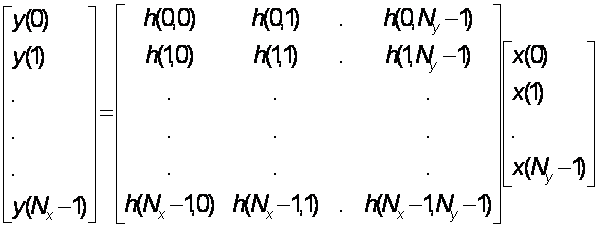Function:

const char* Unfolding(float *source, const float **respMatrix, int ssizex, int ssizey, int numberIterations, int numberRepetitions, double boost)

This function unfolds source spectrum according to response matrix columns. The result is placed in the vector pointed by source pointer.  The coefficients of the resulting vector represent contents of the columns (weights) in the input vector. On successful completion it returns 0. On error it returns pointer to the string describing error. If desired after every numberIterations one can apply boosting operation (exponential function with exponent given by boost coefficient) and repeat it numberRepetitions times. For details we refer to .

Parameters:

source-pointer to the vector of source spectrum

respMatrix-pointer to the matrix of response spectra

ssizex-length of source spectrum and # of columns of the response matrix

ssizey-length of destination spectrum and # of rows of the response matrix

numberIterations-number of iterations

numberRepetitions-number of repetitions for boosted deconvolution. It must be

greater or equal to one.

boost-boosting coefficient, applies only if numberRepetitions is greater than one.

Recommended range <1,2>.

Note!!! sizex must be >= sizey After decomposition the resulting channels are written back to the first sizey channels of the source spectrum.

Reference:

 Jandel M., Morháč M., Kliman J., Krupa L., Matouek V., Hamilton J. H., Ramaya A. V.: Decomposition of continuum gamma-ray spectra using synthetized response matrix. NIM A 516 (2004), 172-183.

```
```

Example of unfolding

Example 13  script Unfolding.c :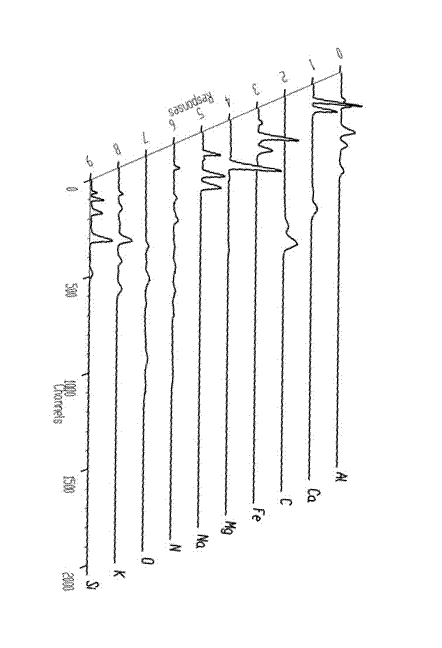Fig. 20  Response matrix composed of neutron spectra of pure chemical elements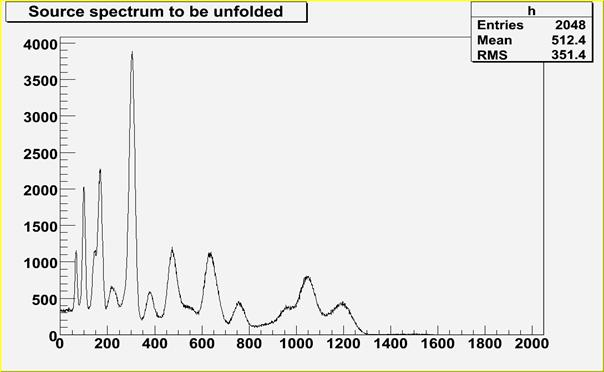Fig. 21 Source neutron spectrum to be decomposed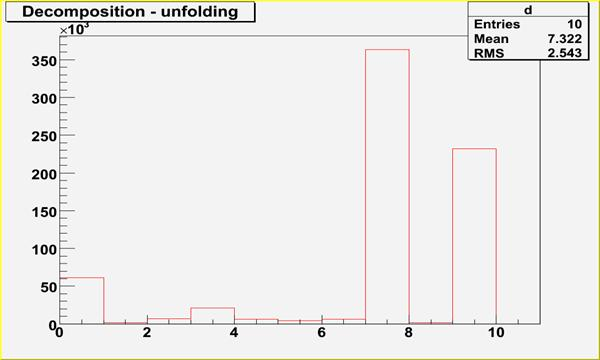Fig. 22 Spectrum after decomposition, contains 10 coefficients, which correspond to contents of chemical components (dominant 8-th and 10-th components, i.e. O, Si)

Script:

// Example to illustrate unfolding function (class TSpectrum).

// To execute this example, do

// root > .x Unfolding.C

#include <TSpectrum>

void Unfolding() {

Int_t i, j;

Int_t nbinsx = 2048;

Int_t nbinsy = 10;

Double_t xmin  = 0;

Double_t xmax  = (Double_t)nbinsx;

Double_t ymin  = 0;

Double_t ymax  = (Double_t)nbinsy;

Float_t * source = new float[nbinsx];

Float_t ** response = new float *[nbinsy];

for (i=0;i<nbinsy;i++)

response[i]=new float[nbinsx];

TH1F *h = new TH1F("h","",nbinsx,xmin,xmax);

TH1F *d = new TH1F("d","Decomposition - unfolding",nbinsx,xmin,xmax);

TH2F *decon_unf_resp = new TH2F("decon_unf_resp","Root File",nbinsy,ymin,ymax,nbinsx,xmin,xmax);

TFile *f = new TFile("spectra\\TSpectrum.root");

h=(TH1F*) f->Get("decon_unf_in;1");

TFile *fr = new TFile("spectra\\TSpectrum.root");

decon_unf_resp = (TH2F*) fr->Get("decon_unf_resp;1");

for (i = 0; i < nbinsx; i++) source[i] = h->GetBinContent(i + 1);

for (i = 0; i < nbinsy; i++){

for (j = 0; j< nbinsx; j++){

response[i][j] = decon_unf_resp->GetBinContent(i + 1, j + 1);

}

}

TCanvas *Decon1 = gROOT->GetListOfCanvases()->FindObject("Decon1");

if (!Decon1) Decon1 = new TCanvas("Decon1","Decon1",10,10,1000,700);

h->Draw("L");

TSpectrum *s = new TSpectrum();

s->Unfolding(source,response,nbinsx,nbinsy,1000,1,1);

for (i = 0; i < nbinsy; i++) d->SetBinContent(i + 1,source[i]);

d->SetLineColor(kRed);

d->SetAxisRange(0,nbinsy);

d->Draw("");

}

Int_t SearchHighRes(float* source, float* destVector, Int_t ssize, float sigma, Double_t threshold, bool backgroundRemove, Int_t deconIterations, bool markov, Int_t averWindow)
```        ONE-DIMENSIONAL HIGH-RESOLUTION PEAK SEARCH FUNCTION
This function searches for peaks in source spectrum
It is based on deconvolution method. First the background is
removed (if desired), then Markov spectrum is calculated
(if desired), then the response function is generated
according to given sigma and deconvolution is carried out.

Function parameters:
source-pointer to the vector of source spectrum
destVector-pointer to the vector of resulting deconvolved spectrum     *
ssize-length of source spectrum
sigma-sigma of searched peaks, for details we refer to manual
threshold-threshold value in % for selected peaks, peaks with
amplitude less than threshold*highest_peak/100
are ignored, see manual
backgroundRemove-logical variable, set if the removal of
background before deconvolution is desired
deconIterations-number of iterations in deconvolution operation
markov-logical variable, if it is true, first the source spectrum
is replaced by new spectrum calculated using Markov
chains method.
averWindow-averanging window of searched peaks, for details
we refer to manual (applies only for Markov method)

```

Peaks searching

Goal: to identify automatically the peaks in spectrum with the presence of the continuous background and statistical fluctuations - noise.

The common problems connected with correct peak identification are

• non-sensitivity to noise, i.e., only statistically relevant peaks should be identified.
• non-sensitivity of the algorithm to continuous background
• ability to identify peaks close to the edges of the spectrum region. Usually peak finders fail to detect them
• resolution, decomposition of doublets and multiplets. The algorithm should be able to recognize close positioned peaks.
• ability to identify peaks with different sigma

Function:

Int_t SearchHighRes(float *source,float *destVector, int ssize, float sigma, double threshold, bool backgroundRemove,int deconIterations, bool markov, int averWindow)

This function searches for peaks in source spectrum. It is based on deconvolution method. First the background is removed (if desired), then Markov smoothed spectrum is calculated (if desired), then the response function is generated according to given sigma and deconvolution is carried out. The order of peaks is arranged according to their heights in the spectrum after background elimination. The highest peak is the first in the list. On success it returns number of found peaks.

Parameters:

source-pointer to the vector of source spectrum

destVector-resulting spectrum after deconvolution

ssize-length of the source and destination spectra

sigma-sigma of searched peaks

threshold- threshold value in % for selected peaks, peaks with amplitude less than threshold*highest_peak/100 are ignored

backgroundRemove- background_remove-logical variable, true if the removal of background before deconvolution is desired

deconIterations-number of iterations in deconvolution operation

markov-logical variable, if it is true, first the source spectrum is replaced by new spectrum calculated using Markov chains method

averWindow-width of averaging smoothing windowFig. 27 An example of one-dimensional synthetic spectrum with found peaks denoted by markers

References:

 M.A. Mariscotti: A method for identification of peaks in the presence of background and its application to spectrum analysis. NIM 50 (1967), 309-320.

  M. Morháč, J. Kliman, V. Matouek, M. Veselský, I. Turzo.:Identification of peaks in multidimensional coincidence gamma-ray spectra. NIM, A443 (2000) 108-125.

 Z.K. Silagadze, A new algorithm for automatic photopeak searches. NIM A 376 (1996), 451.

```
```

Examples of peak searching method

SearchHighRes function provides users with the possibility to vary the input parameters and with the access to the output deconvolved data in the destination spectrum. Based on the output data one can tune the parameters.

Example 15  script SearchHR1.c:Fig. 28 One-dimensional spectrum with found peaks denoted by markers, 3 iterations steps in the deconvolution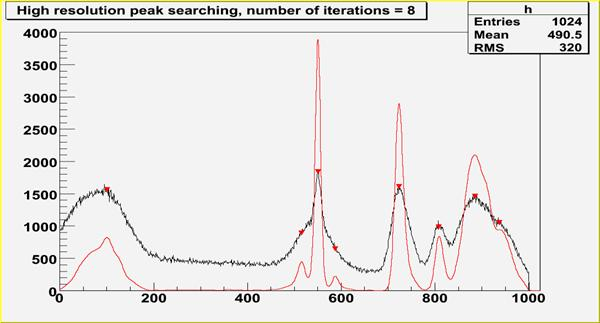Fig. 29 One-dimensional spectrum with found peaks denoted by markers, 8 iterations steps in the deconvolution

Script:

// Example to illustrate high resolution peak searching function (class TSpectrum).

// To execute this example, do

// root > .x SearchHR1.C

#include <TSpectrum>

void SearchHR1() {

Float_t fPositionX;

Float_t fPositionY;

Int_t fNPeaks = 0;

Int_t i,nfound,bin;

Double_t nbins = 1024,a;

Double_t xmin  = 0;

Double_t xmax  = (Double_t)nbins;

Float_t * source = new float[nbins];

Float_t * dest = new float[nbins];

TH1F *h = new TH1F("h","High resolution peak searching, number of iterations = 3",nbins,xmin,xmax);

TH1F *d = new TH1F("d","",nbins,xmin,xmax);

TFile *f = new TFile("spectra\\TSpectrum.root");

h=(TH1F*) f->Get("search2;1");

for (i = 0; i < nbins; i++) source[i]=h->GetBinContent(i + 1);

TCanvas *Search = gROOT->GetListOfCanvases()->FindObject("Search");

if (!Search) Search = new TCanvas("Search","Search",10,10,1000,700);

h->SetMaximum(4000);

h->Draw("L");

TSpectrum *s = new TSpectrum();

nfound = s->SearchHighRes(source, dest, nbins, 8, 2, kTRUE, 3, kTRUE, 3);

Float_t *xpeaks = s->GetPositionX();

for (i = 0; i < nfound; i++) {

a=xpeaks[i];

bin = 1 + Int_t(a + 0.5);

fPositionX[i] = h->GetBinCenter(bin);

fPositionY[i] = h->GetBinContent(bin);

}

TPolyMarker * pm = (TPolyMarker*)h->GetListOfFunctions()->FindObject("TPolyMarker");

if (pm) {

h->GetListOfFunctions()->Remove(pm);

delete pm;

}

pm = new TPolyMarker(nfound, fPositionX, fPositionY);

pm->SetMarkerStyle(23);

pm->SetMarkerColor(kRed);

pm->SetMarkerSize(1.3);

for (i = 0; i < nbins; i++) d->SetBinContent(i + 1,dest[i]);

d->SetLineColor(kRed);

d->Draw("SAME");

printf("Found %d candidate peaks\n",nfound);

for(i=0;i<nfound;i++)

printf("posx= %d, posy= %d\n",fPositionX[i], fPositionY[i]);

}

Example 16  script SearchHR3.c:

 Peak # Position Sigma 1 118 26 2 162 41 3 310 4 4 330 8 5 482 22 6 491 26 7 740 21 8 852 15 9 954 12 10 989 13

Table 4 Positions and sigma of peaks in the following examples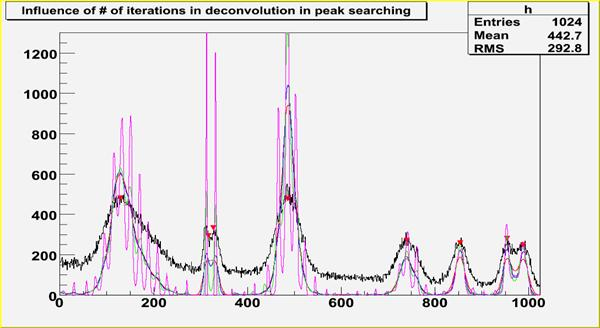Fig. 30 Influence of number of iterations (3-red, 10-blue, 100- green, 1000-magenta), sigma=8, smoothing width=3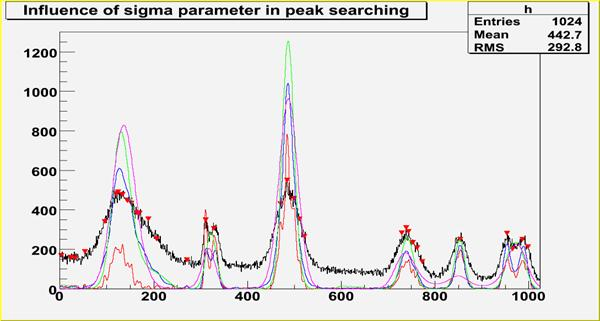Fig. 31 Influence of sigma (3-red, 8-blue, 20- green, 43-magenta), num. iter.=10, sm. width=3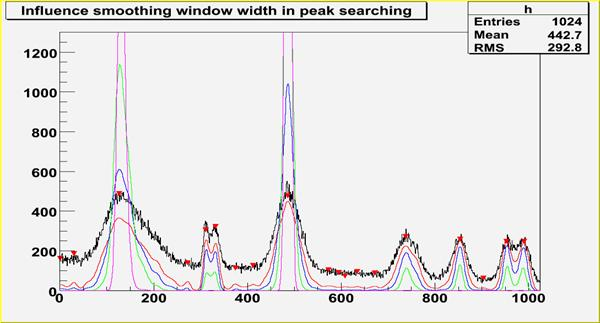Fig. 32 Influence smoothing width (0-red, 3-blue, 7- green, 20-magenta), num. iter.=10, sigma=8

Script:

// Example to illustrate the influence of number of iterations in deconvolution in high resolution peak searching function (class TSpectrum).

// To execute this example, do

// root > .x SearchHR3.C

#include <TSpectrum>

void SearchHR3() {

Float_t fPositionX;

Float_t fPositionY;

Int_t fNPeaks = 0;

Int_t i,nfound,bin;

Double_t nbins = 1024,a;

Double_t xmin  = 0;

Double_t xmax  = (Double_t)nbins;

Float_t * source = new float[nbins];

Float_t * dest = new float[nbins];

TH1F *h = new TH1F("h","Influence of # of iterations in deconvolution in peak searching",nbins,xmin,xmax);

TH1F *d1 = new TH1F("d1","",nbins,xmin,xmax);

TH1F *d2 = new TH1F("d2","",nbins,xmin,xmax);

TH1F *d3 = new TH1F("d3","",nbins,xmin,xmax);

TH1F *d4 = new TH1F("d4","",nbins,xmin,xmax);

TFile *f = new TFile("spectra\\TSpectrum.root");

h=(TH1F*) f->Get("search3;1");

for (i = 0; i < nbins; i++) source[i]=h->GetBinContent(i + 1);

TCanvas *Search = gROOT->GetListOfCanvases()->FindObject("Search");

if (!Search) Search = new TCanvas("Search","Search",10,10,1000,700);

h->SetMaximum(1300);

h->Draw("L");

TSpectrum *s = new TSpectrum();

nfound = s->SearchHighRes(source, dest, nbins, 8, 2, kTRUE, 3, kTRUE, 3);

Float_t *xpeaks = s->GetPositionX();

for (i = 0; i < nfound; i++) {

a=xpeaks[i];

bin = 1 + Int_t(a + 0.5);

fPositionX[i] = h->GetBinCenter(bin);

fPositionY[i] = h->GetBinContent(bin);

}

TPolyMarker * pm = (TPolyMarker*)h->GetListOfFunctions()->FindObject("TPolyMarker");

if (pm) {

h->GetListOfFunctions()->Remove(pm);

delete pm;

}

pm = new TPolyMarker(nfound, fPositionX, fPositionY);

pm->SetMarkerStyle(23);

pm->SetMarkerColor(kRed);

pm->SetMarkerSize(1.3);

for (i = 0; i < nbins; i++) d1->SetBinContent(i + 1,dest[i]);

h->Draw("");

d1->SetLineColor(kRed);

d1->Draw("SAME");

for (i = 0; i < nbins; i++) source[i]=h->GetBinContent(i + 1);

s->SearchHighRes(source, dest, nbins, 8, 2, kTRUE, 10, kTRUE, 3);

for (i = 0; i < nbins; i++) d2->SetBinContent(i + 1,dest[i]);

d2->SetLineColor(kBlue);

d2->Draw("SAME");

for (i = 0; i < nbins; i++) source[i]=h->GetBinContent(i + 1);

s->SearchHighRes(source, dest, nbins, 8, 2, kTRUE, 100, kTRUE, 3);

for (i = 0; i < nbins; i++) d3->SetBinContent(i + 1,dest[i]);

d3->SetLineColor(kGreen);

d3->Draw("SAME");

for (i = 0; i < nbins; i++) source[i]=h->GetBinContent(i + 1);

s->SearchHighRes(source, dest, nbins, 8, 2, kTRUE, 1000, kTRUE, 3);

for (i = 0; i < nbins; i++) d4->SetBinContent(i + 1,dest[i]);

d4->SetLineColor(kMagenta);

d4->Draw("SAME");

printf("Found %d candidate peaks\n",nfound);

}

Int_t Search1HighRes(float* source, float* destVector, Int_t ssize, float sigma, Double_t threshold, bool backgroundRemove, Int_t deconIterations, bool markov, Int_t averWindow)
```  Old name of SearcHighRes introduced for back compatibility
This function will be removed after the June 2006 release
```
Int_t StaticSearch(const TH1* hist, Double_t sigma = 2, Option_t* option = "goff", Double_t threshold = 0.05)
```static function, interface to TSpectrum::Search
```
TH1 * StaticBackground(const TH1* hist, Int_t niter = 20, Option_t* option = "")
```static function, interface to TSpectrum::Background
```
TSpectrum(const TSpectrum& )
TSpectrum& operator=(const TSpectrum& )

`{return fHistogram;}`

`{return fNPeaks;}`

`{return fPositionX;}`

`{return fPositionY;}`

Author: Miroslav Morhac 27/05/99
Last update: root/spectrum:\$Id: TSpectrum.h 20882 2007-11-19 11:31:26Z rdm \$